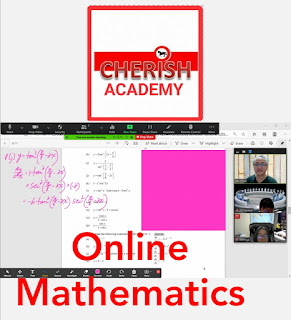## Thursday, November 10, 2011

### Master My Maths 2016 Maths Programme

3AM & 4AM Programme . Call us

Binomial Theorem
Equation of circle
Geometry Proof
Trigonometry Function
Trigonometry Graph
Trigonometry Identities
Trigonometry Equations 1& 11
Differentiation and Techniques- product rule / quotient rule
Differentiation of trigonometry Functions
Differentiation of exponential and Logarithm Functions
Differentiation and  Rate of Change
Differentiation - maximum / minimum point
Differentiation & application
Differentiation - kinematics
Integration
Integration on trigonometry
Integration on exponential power
Integration on application
Integration - area
Integration - kinematic

3AM Master My Maths
Factorization
Algebraic Expansion
Simple indices
Simultaneous Equations
Remainder Theorems
Factor Theorem
Indices
Surds
Inequalities
Roots of equations
Partial Fractions
Exponential Equations
Logarithm
Modulus Function
Coordinate Geometry
Linear law
Binomial theorems
Equations of Circle